WPS Office

Free All-in-One Office Suite with PDF Editor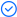Read, edit, and convert PDFs with the powerful PDF toolkit.Microsoft-like interface, easy to use.

Windows • MacOS • Linux • iOS • Android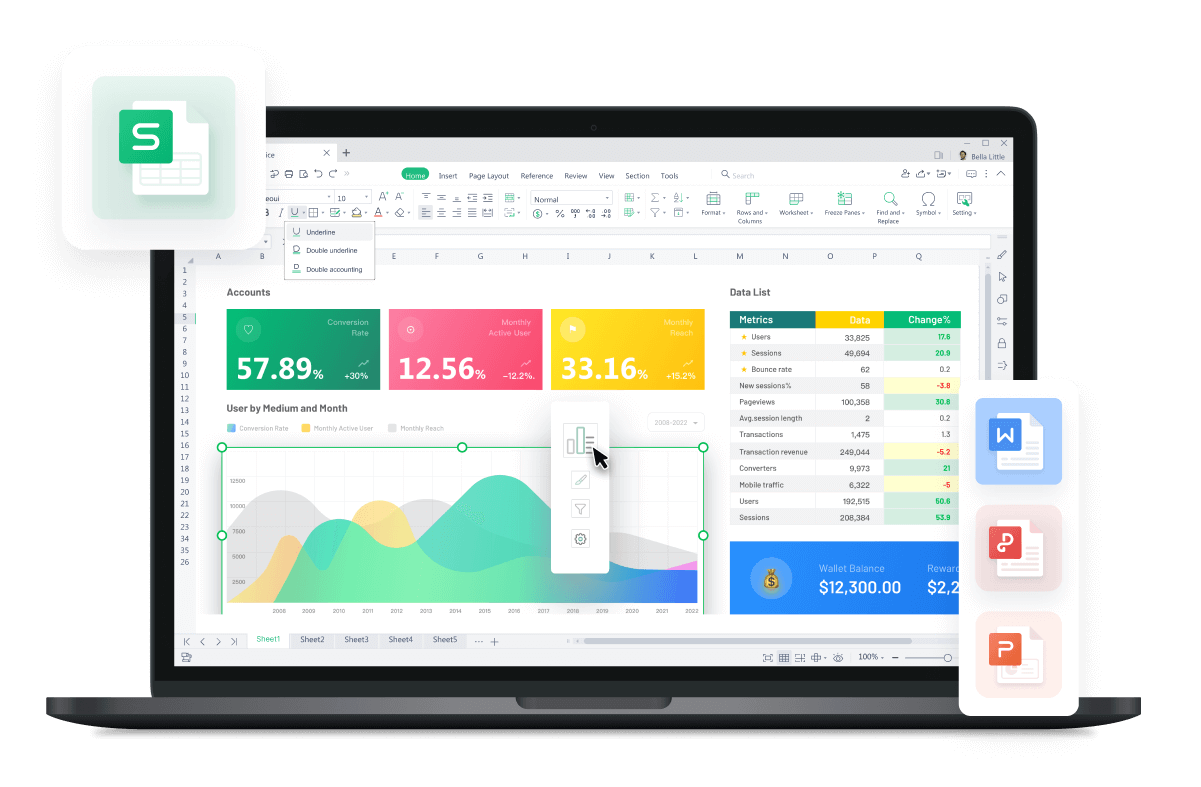# Continuous Compounding Formula in Excel

August 9, 2022
6.3K Views

The continuous compounding formula is mandatory to calculate the growth of an investment or the accumulation of interest when compounding occurs continuously over time. It is useful in financial calculations and investment analysis, enabling more accurate projections and optimizing investment strategies.

While doing statistical analysis, sometimes we cannot figure out the present value, which is where the Continuous compounding formula kicks in. This guide will help you understand How to calculate current value continuous compounding in Excel, so stick until the end.

## Method-1 How to use continuous compounding formula in Excel

1. Open an Excel spreadsheet on your PC and select the cell where you want to calculate the present value (PV).2.

2. To find the present value, we must first input the following parameters. These parameters include

• Interest rate (Rate)

• Number of Payment periods (Nper)

• Payment made in each period. It must be negative (Pmt)

• Present value of future payments. If no value is assigned to it, then Excel takes it as zero (PV)

• When Payments are due. 1 = beginning of the period and 0 = end of the period. (Type)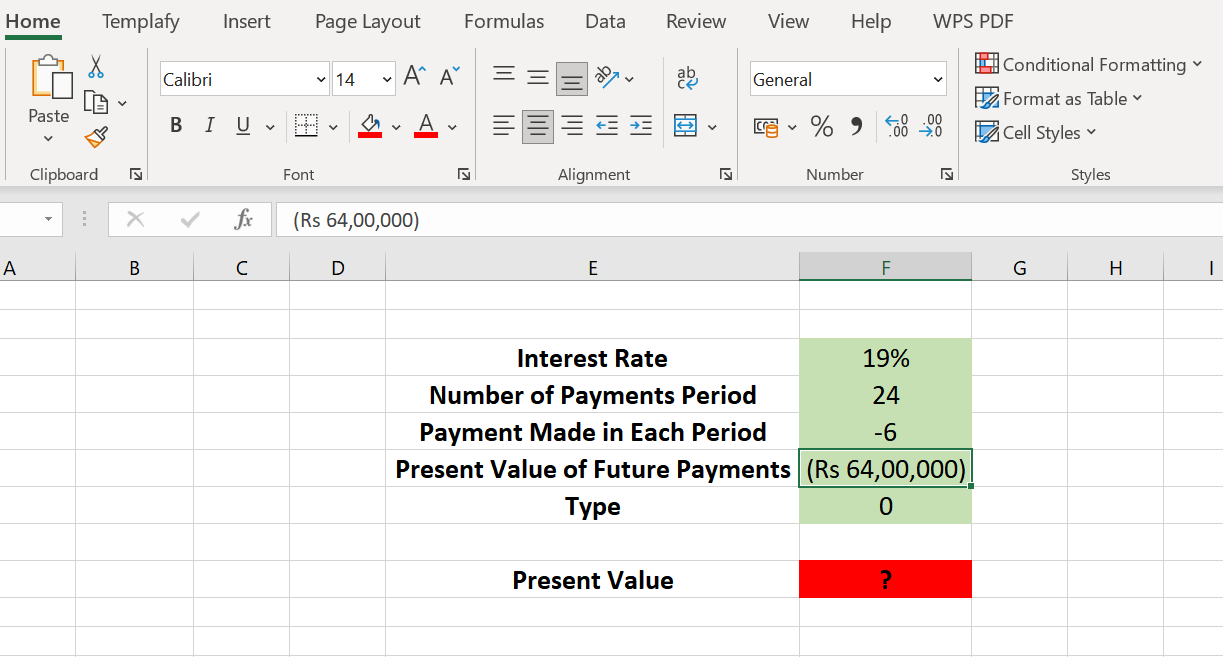3. Once all inputs are provided, click the Formulas tab and select Insert Function.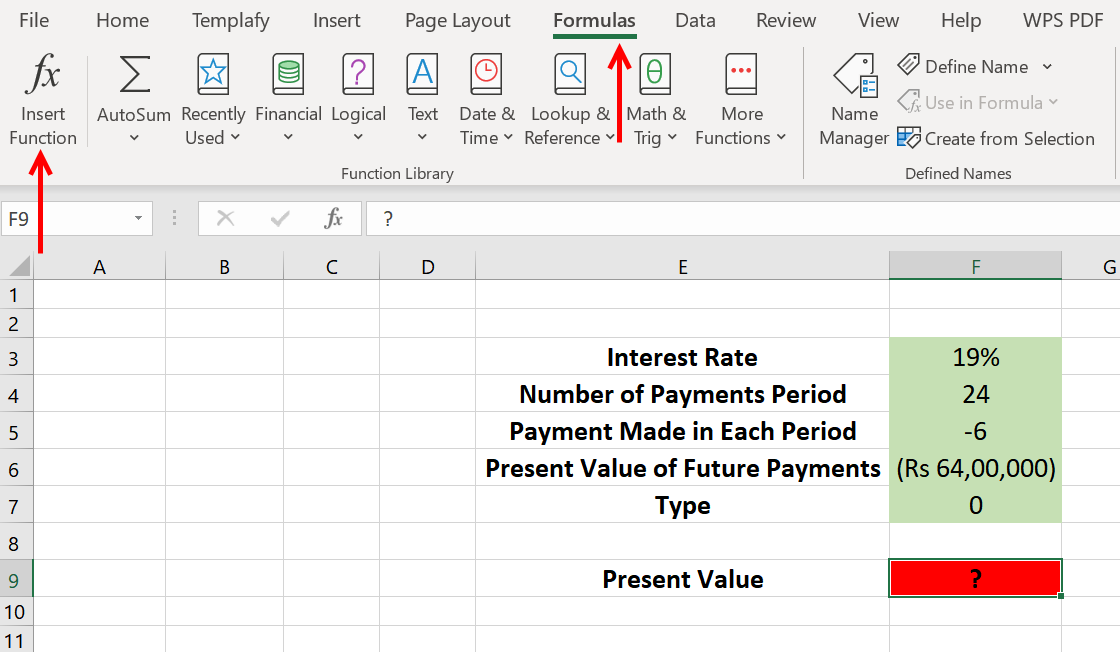4. It will open a list of functions. Type PV in the search, click GO, select PV from the list, and click Ok.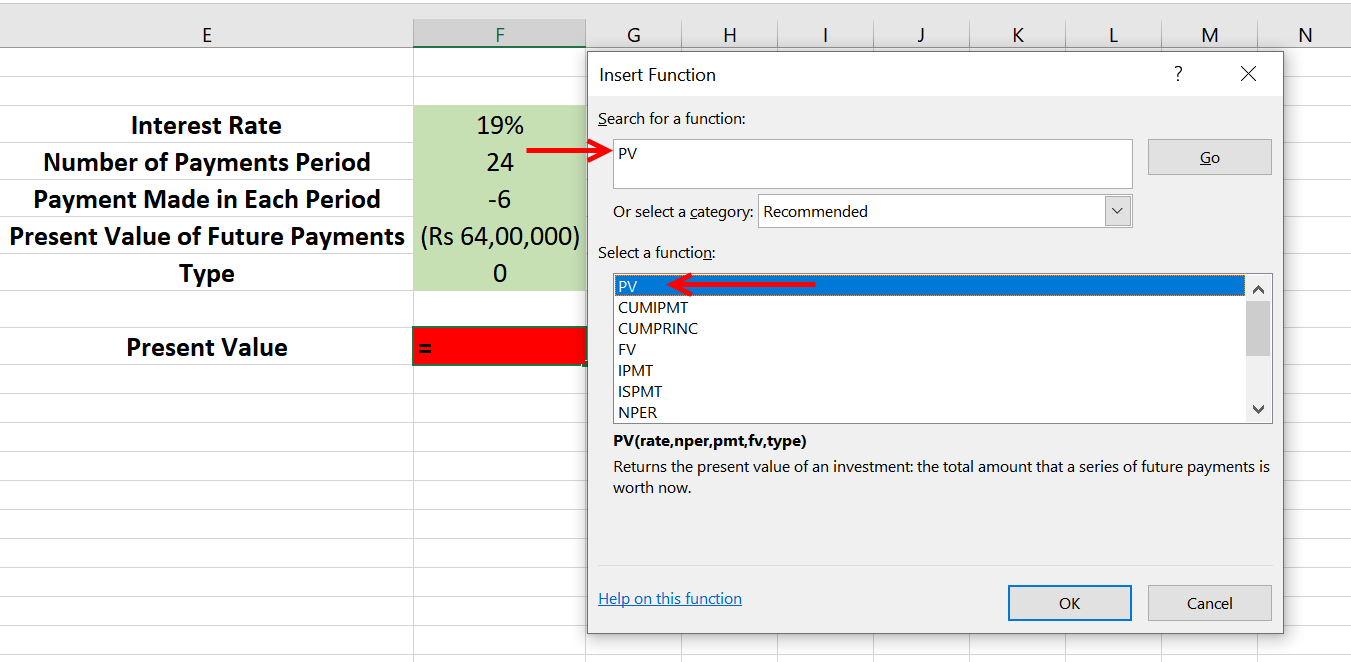5. It will open a list of inputs you recently provided but with reference to its cells. Simply click on the input tab and then select the appropriate cell.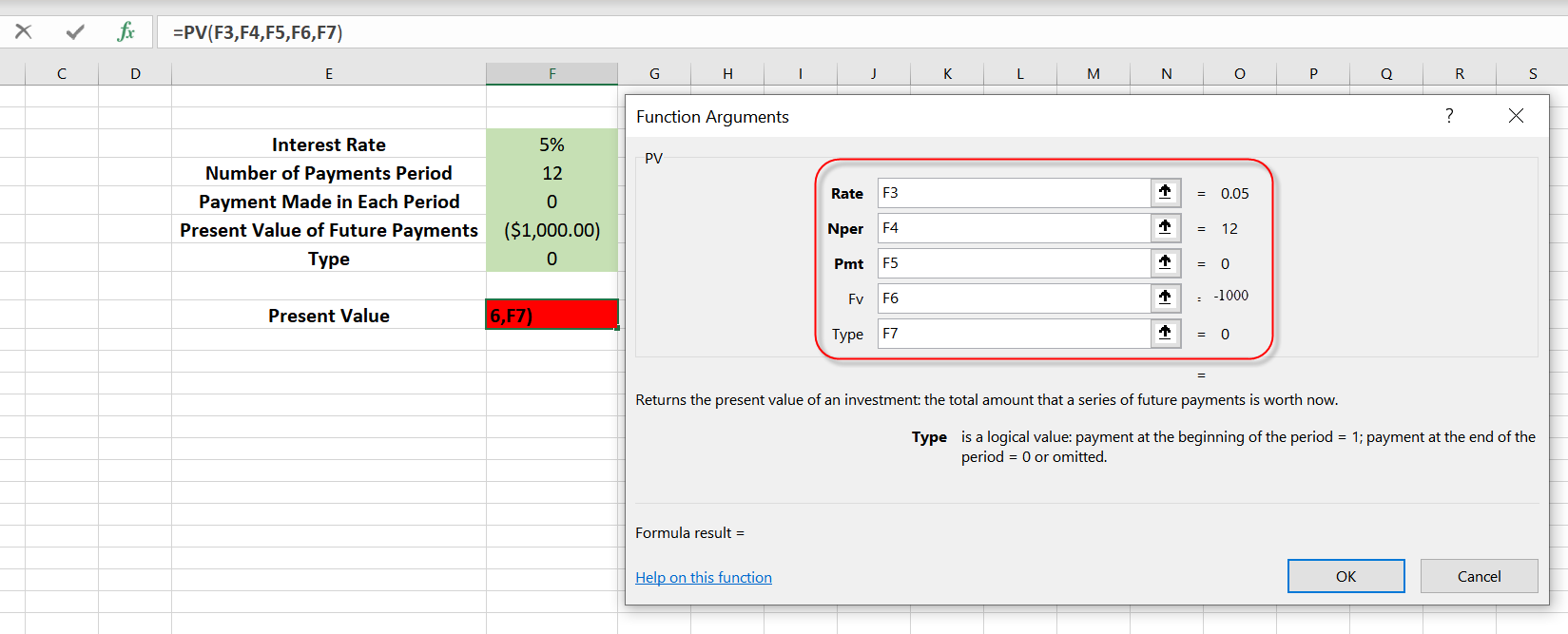6. It will give you the output for PV, as shown below.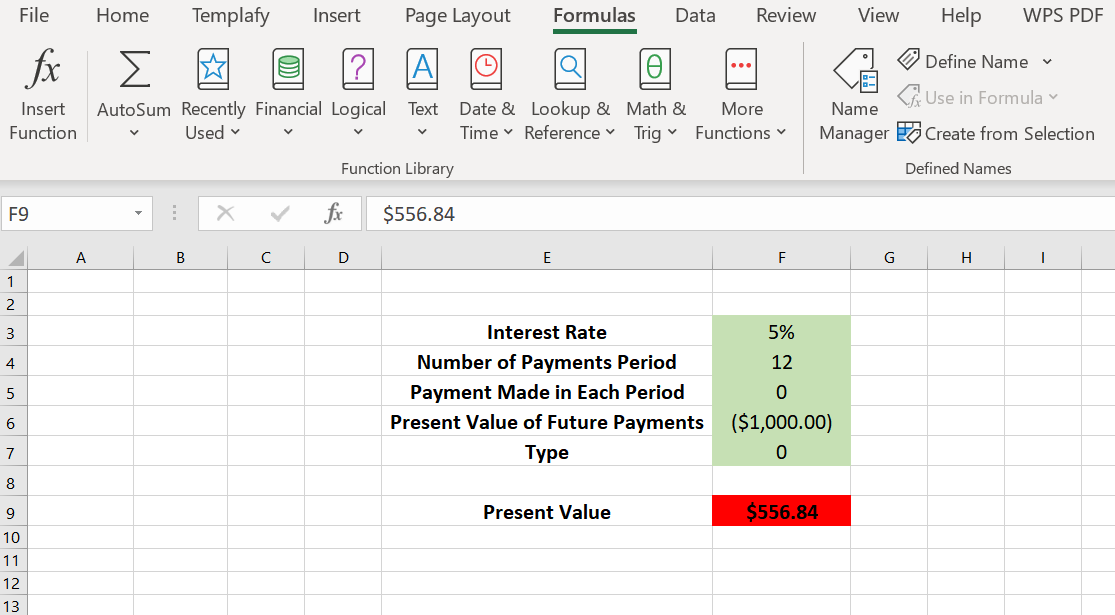7. Now we have the PV and can use the Excel Continuous Compounding formula.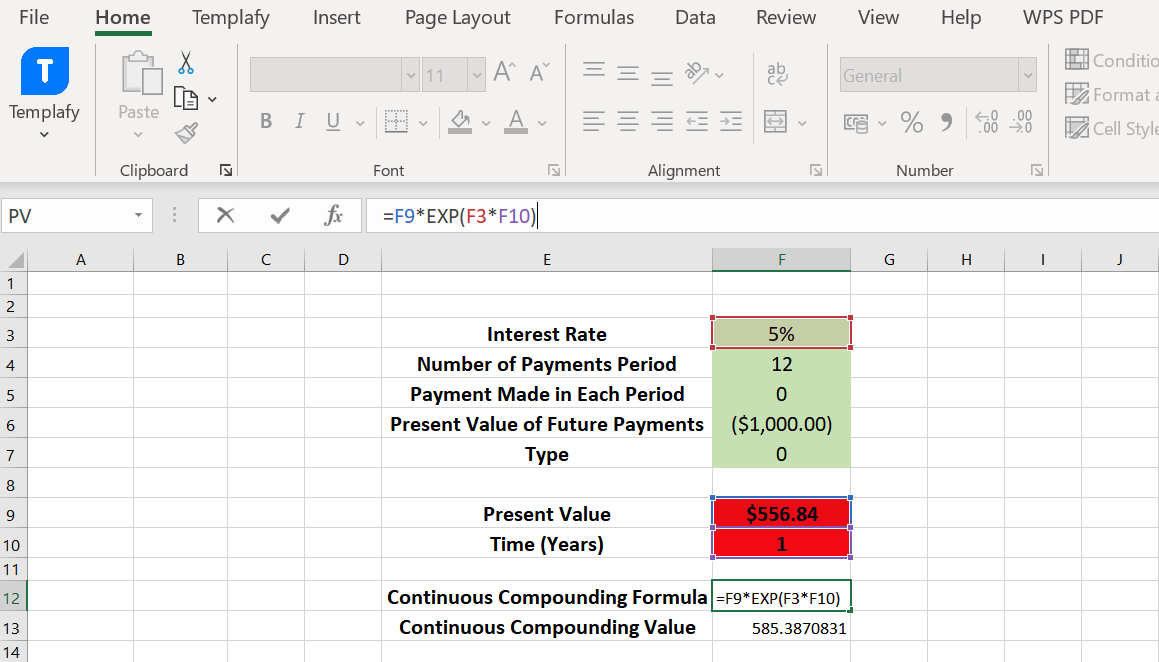## Method-2 To use continuous compounding formula as an investment in Excel.

By setting the payment argument to zero, you can use the FV function to calculate the future value of a lump sum investment with continuous compounding. The syntax for the FV function is as follows:

=FV(rate, nper, [pmt], [pv], [type])

• Open a new or existing Excel spreadsheet.

• Decide on the values you want to use for the present value (PV), annual interest rate (r), and number of years (t) in your calculation.

• In a cell where you want to display the future value of the investment, enter the following formula: =FV(r, t, 0, PV)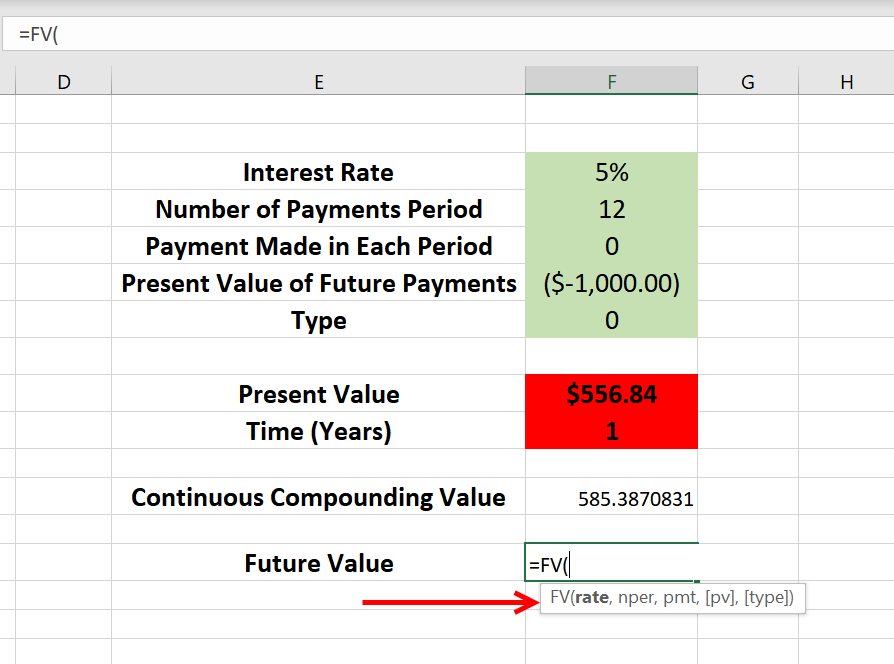• Replace "r," "t," and "PV" in the formula with the cell references or the actual values you want to use.

• For example, if your annual interest rate is in cell A2, the number of years is in cell A3, and the present value is in cell A1, the formula would be: =FV(F3, F4, 0, F9)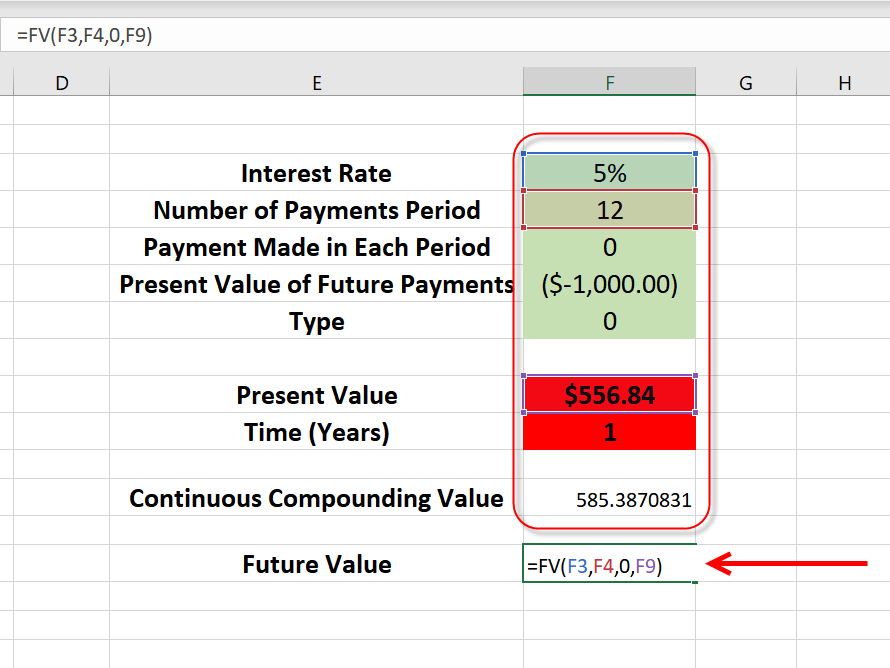• Press Enter to calculate the future value of the investment with continuous compounding.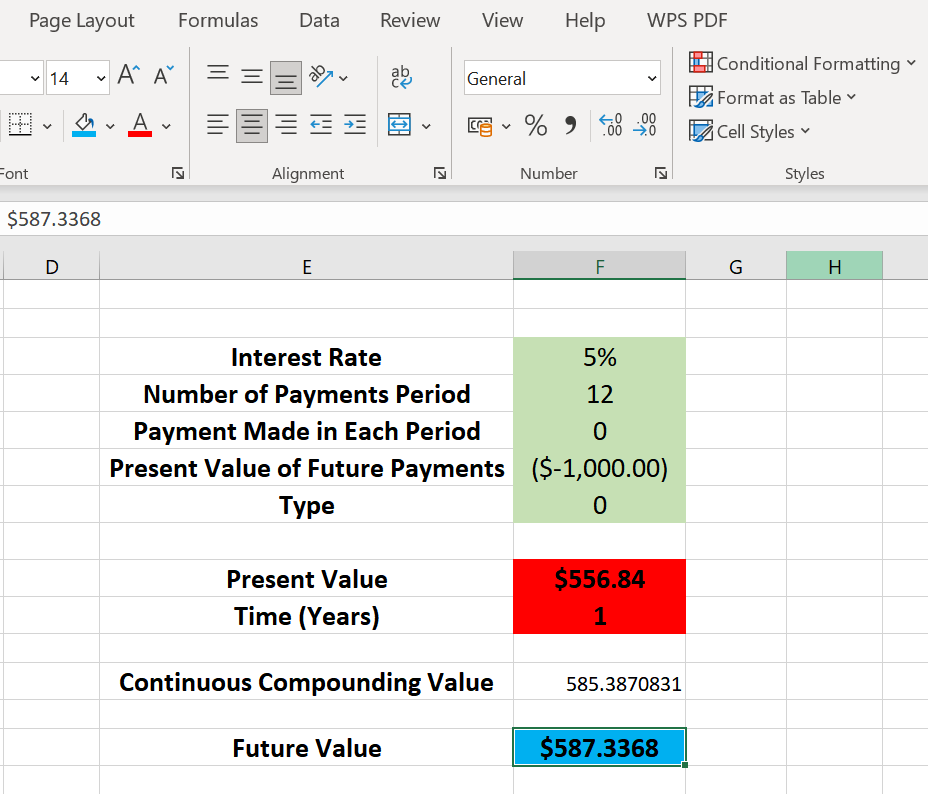## Different uses for using continuous compounding formulas in Excel

The continuous compound formula is used for multiple purposes and primarily by investors, business owners, and financial analysts to calculate the growth of an investment.

### For Investors

Investors can use this formula to calculate the future value of an investment with continuous compounding, which can help them decide where to invest their money. It enables them to understand the impact of compounding on their returns and make informed decisions regarding investment strategies and expected future values.

For example, investors can use the formula to estimate the future value of savings accounts, calculate the growth of investment portfolios, or compare the potential returns of different investment options.

### For Financial Analysts

Financial analysts can use this formula to analyze investment opportunities and build economic models. They may use it to calculate the present value of an investment, forecast future earnings, or determine the appropriate discount rate to use in a valuation model. They can model the growth of assets, estimate future values, and evaluate the potential returns of various financial instruments or investment opportunities.

They also use it to perform present value calculations, assess the profitability of projects, conduct valuation analyses, and make recommendations to clients or management based on the projected growth or value of investments.

Business owners can benefit from the continuous compounding formula when making financial decisions and evaluating the financial performance of their companies. They can also use it to calculate the future value of an investment made by the business, such as a new piece of equipment or a new product line. They can make informed decisions about funding options, expansion plans, or investment strategies by leveraging the continuous compounding formula in Excel.

## FAQs about using continuous compounding formulas in Excel

### Q1. How accurate is this continuous compounding formula?

The continuous compounding formula assumes that interest is added to the principal every instant. Interest is typically calculated and added at discrete intervals such as daily, monthly, or annually. While continuous compounding can provide a more precise estimate of future value, it may only sometimes be practical or necessary.

### Q2. How complex is this continuous compounding formula?

The continuous compounding formula involves a complex mathematical calculation that some users may need help understanding or applying. It requires knowledge of exponential functions and logarithms, which may be familiar to some. But once you know the basic components, using the formula becomes easier.

### Q3. Would using this continuous compounding formula cause error?

Properly using the continuous compounding formula should avoid errors. However, as with any formula, incorrect inputs or misuse can lead to inaccurate results. It's important to consistently ensure accurate P, r, and t values and apply the formula. Careful attention to detail and validation of inputs help avoid errors and provide accurate calculations.

### Q4. Would using this continuous compounding formula cause an unrealistic problem?

The continuous compounding formula assumes that interest rates remain constant over time, which may not be true in real-world situations. It also takes that there are no taxes or fees associated with the investment, which may not be accurate. Realistic or accurate interest rate values, periods, or initial amounts can lead to practical outcomes.

## Summary

The compound interest calculator is handy for investors, business owners, and financial analysts to calculate the future value of an investment when interest is compounded continuously. It depends on multiple parameters, and they decide the result of the formula. The methods mentioned above are straightforward and can be used to calculate future investment returns. Moreover, these methods are also applicable to WPS Office.

WPS Office is a free alternative to MS Office, packed with essential softwares, including spreadsheets, word processing, presentation, and a potent PDF Tool. It is a lightweight version of MS Office and reverse compatible with MS Office, so you can work on different softwares using the same files. Installing it on your PC is highly recommended if you haven’t tried WPS Office yet.